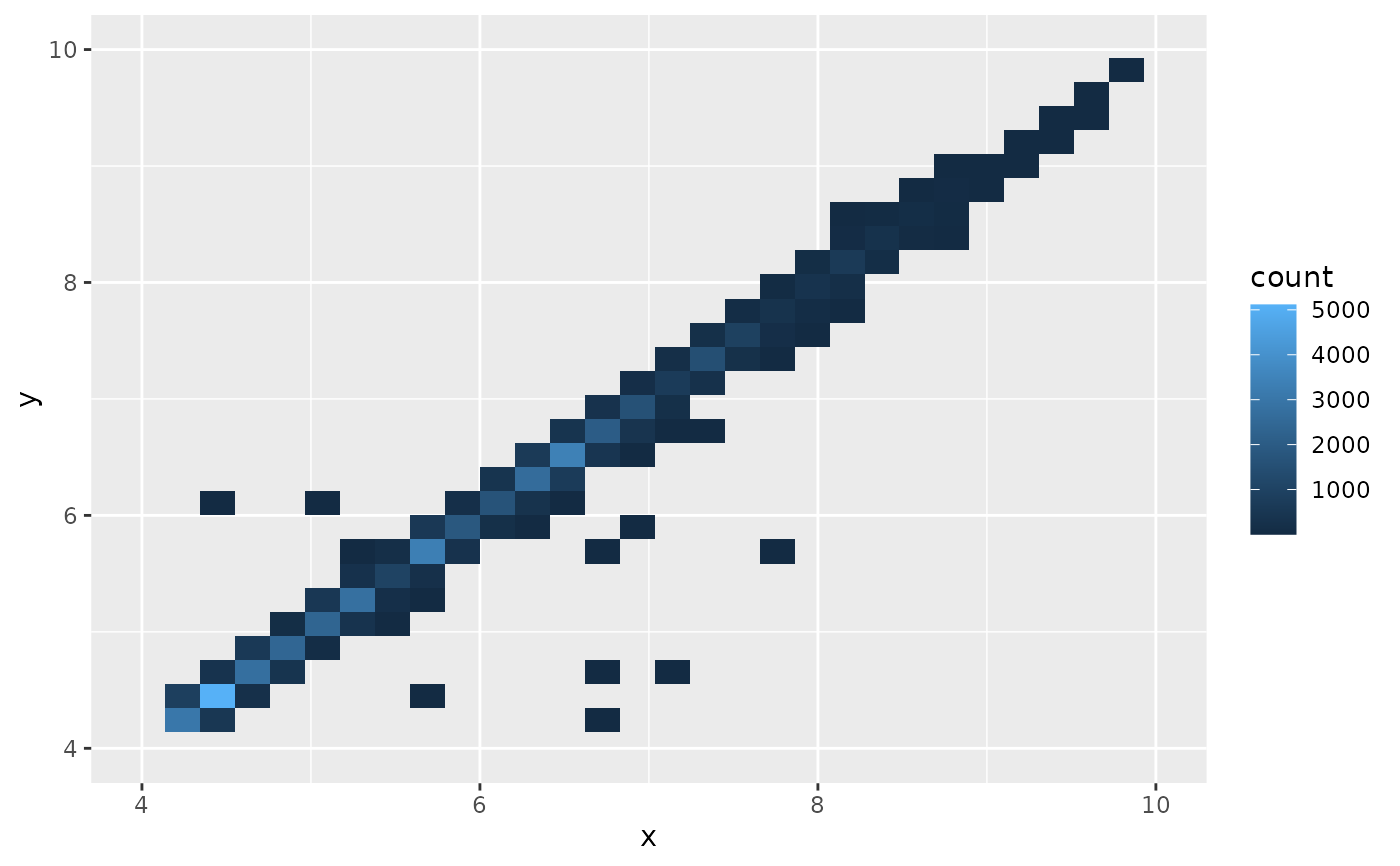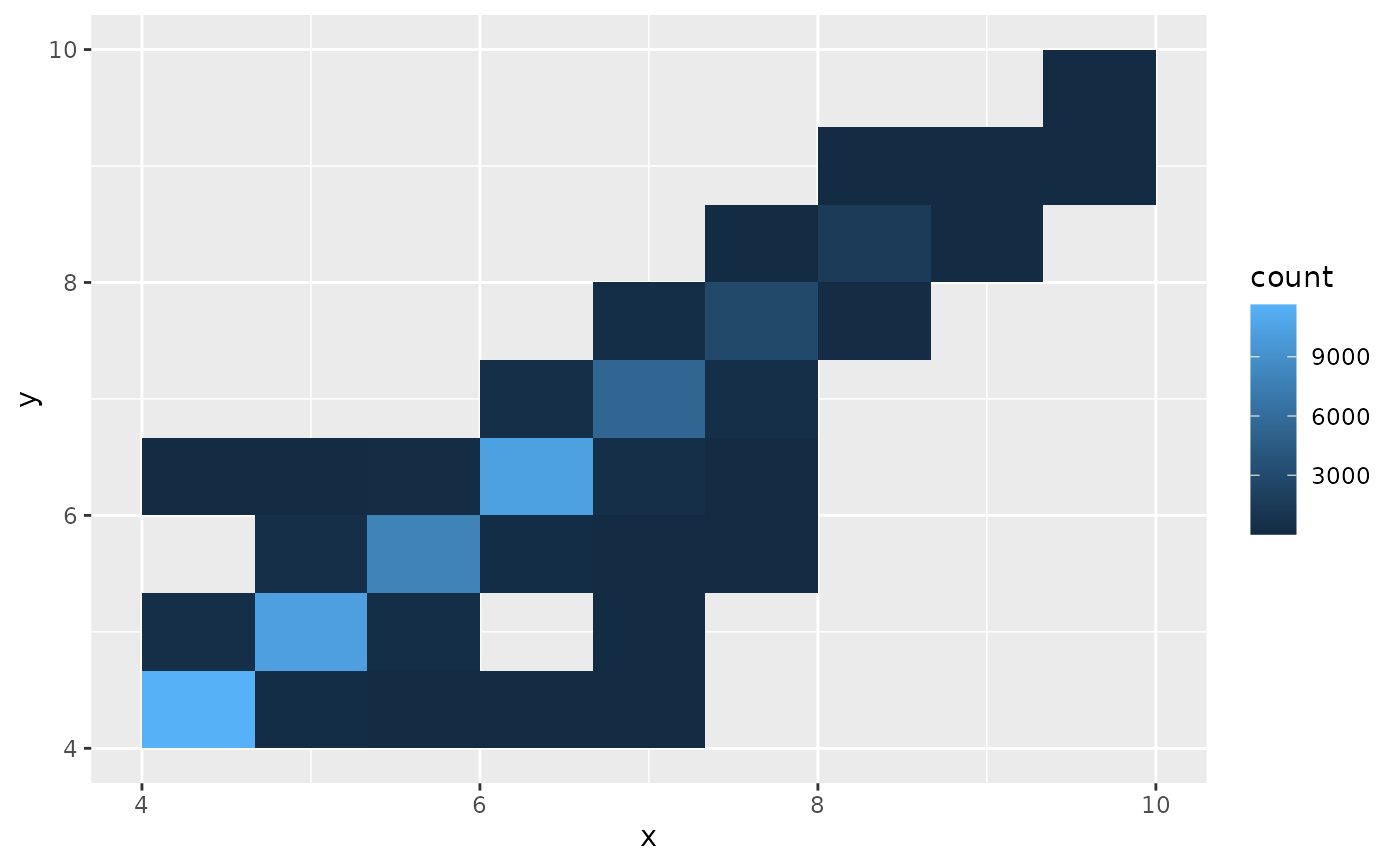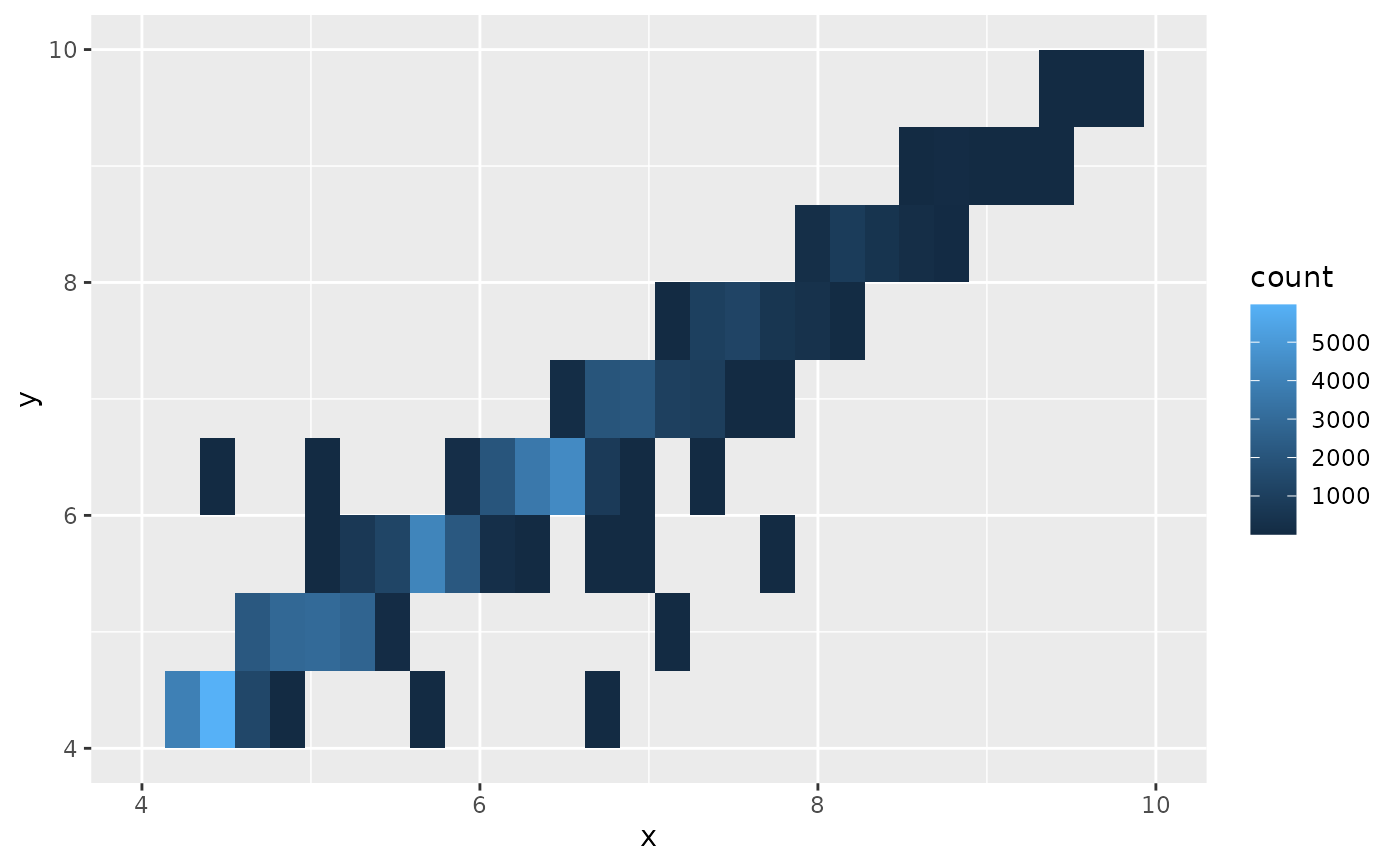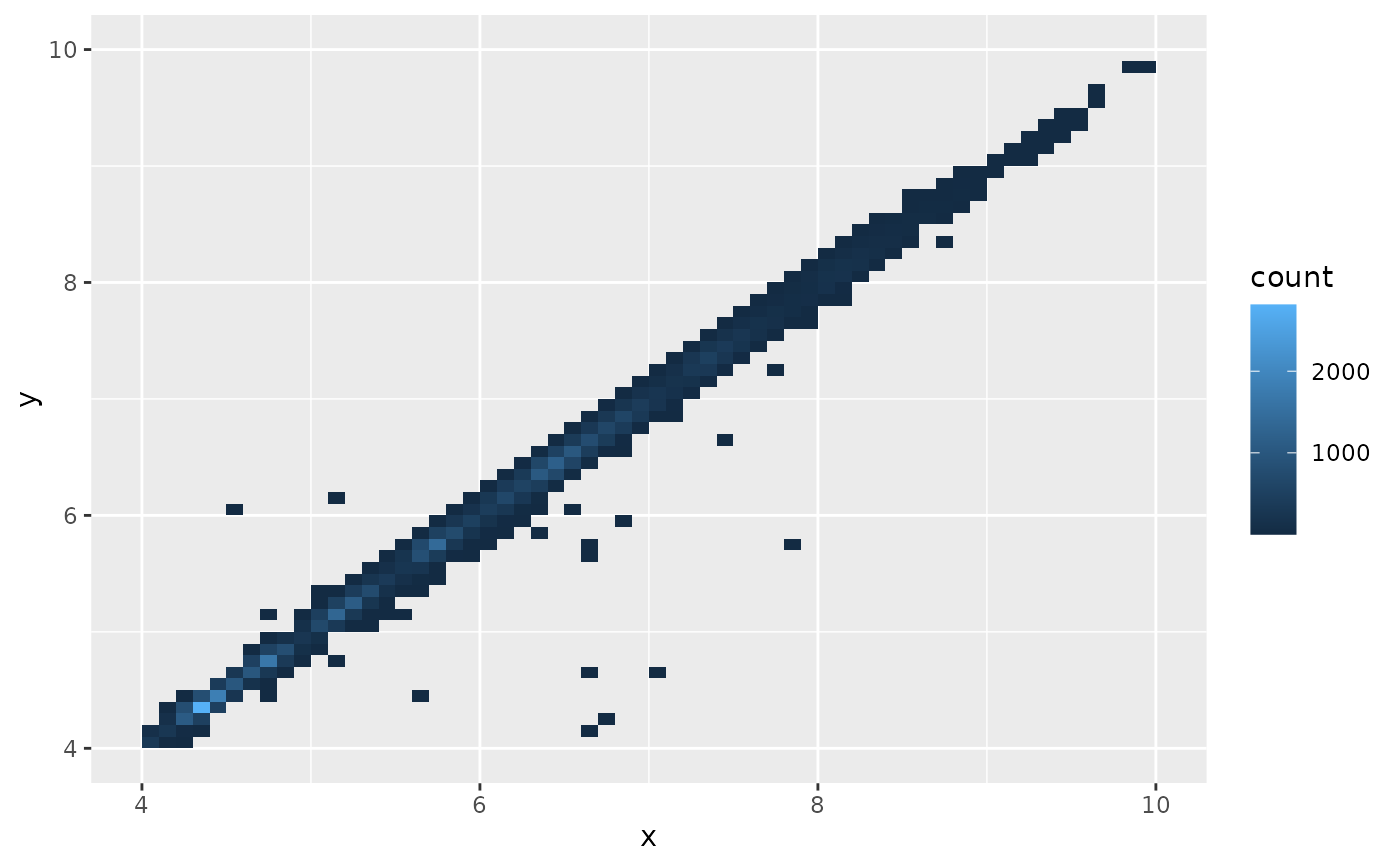Divides the plane into rectangles, counts the number of cases in each rectangle, and then (by default) maps the number of cases to the rectangle's fill. This is a useful alternative to geom_point() in the presence of overplotting.

geom_bin_2d(
mapping = NULL,
data = NULL,
stat = "bin2d",
position = "identity",
...,
na.rm = FALSE,
show.legend = NA,
inherit.aes = TRUE
)

stat_bin_2d(
mapping = NULL,
data = NULL,
geom = "tile",
position = "identity",
...,
bins = 30,
binwidth = NULL,
drop = TRUE,
na.rm = FALSE,
show.legend = NA,
inherit.aes = TRUE
)

## Arguments

mapping Set of aesthetic mappings created by aes() or aes_(). If specified and inherit.aes = TRUE (the default), it is combined with the default mapping at the top level of the plot. You must supply mapping if there is no plot mapping. The data to be displayed in this layer. There are three options: If NULL, the default, the data is inherited from the plot data as specified in the call to ggplot(). A data.frame, or other object, will override the plot data. All objects will be fortified to produce a data frame. See fortify() for which variables will be created. A function will be called with a single argument, the plot data. The return value must be a data.frame, and will be used as the layer data. A function can be created from a formula (e.g. ~ head(.x, 10)). Position adjustment, either as a string, or the result of a call to a position adjustment function. Other arguments passed on to layer(). These are often aesthetics, used to set an aesthetic to a fixed value, like colour = "red" or size = 3. They may also be parameters to the paired geom/stat. If FALSE, the default, missing values are removed with a warning. If TRUE, missing values are silently removed. logical. Should this layer be included in the legends? NA, the default, includes if any aesthetics are mapped. FALSE never includes, and TRUE always includes. It can also be a named logical vector to finely select the aesthetics to display. If FALSE, overrides the default aesthetics, rather than combining with them. This is most useful for helper functions that define both data and aesthetics and shouldn't inherit behaviour from the default plot specification, e.g. borders(). Use to override the default connection between geom_bin_2d() and stat_bin_2d(). numeric vector giving number of bins in both vertical and horizontal directions. Set to 30 by default. Numeric vector giving bin width in both vertical and horizontal directions. Overrides bins if both set. if TRUE removes all cells with 0 counts.

## Aesthetics

stat_bin2d() understands the following aesthetics (required aesthetics are in bold):

• x

• y

• fill

• group

Learn more about setting these aesthetics in vignette("ggplot2-specs").

## Computed variables

count

number of points in bin

density

density of points in bin, scaled to integrate to 1

ncount

count, scaled to maximum of 1

ndensity

density, scaled to maximum of 1

stat_binhex() for hexagonal binning

## Examples

d <- ggplot(diamonds, aes(x, y)) + xlim(4, 10) + ylim(4, 10)
d + geom_bin_2d()
#> Warning: Removed 478 rows containing non-finite values (stat_bin2d).# You can control the size of the bins by specifying the number of
# bins in each direction:
d + geom_bin_2d(bins = 10)
#> Warning: Removed 478 rows containing non-finite values (stat_bin2d).
#> Warning: Removed 4 rows containing missing values (geom_tile).d + geom_bin_2d(bins = 30)
#> Warning: Removed 478 rows containing non-finite values (stat_bin2d).# Or by specifying the width of the bins
d + geom_bin_2d(binwidth = c(0.1, 0.1))
#> Warning: Removed 478 rows containing non-finite values (stat_bin2d).